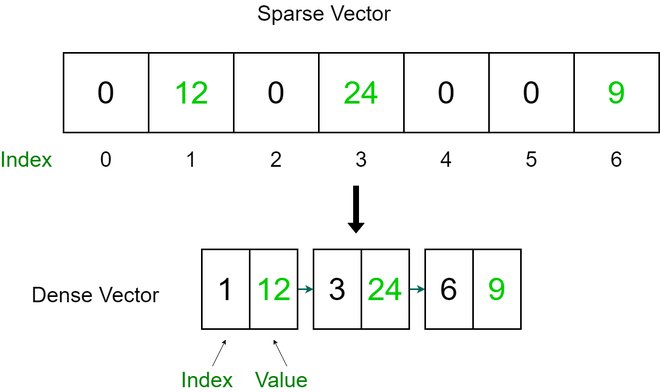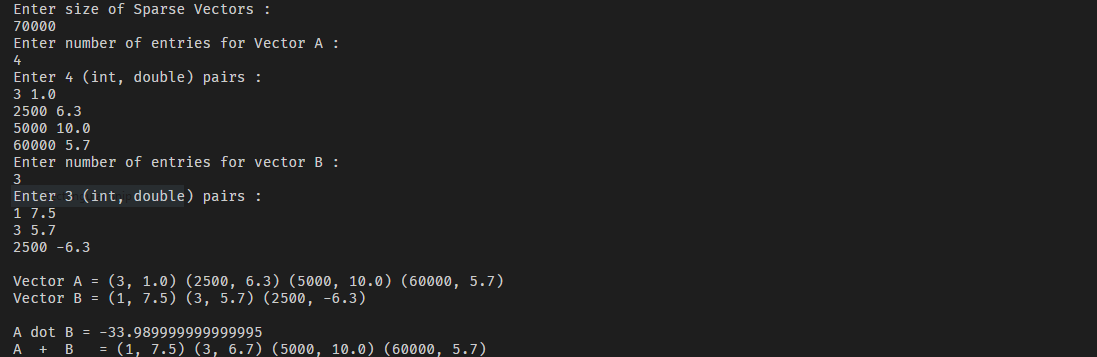Skip to content
Related Articles
Implementing Sparse Vector in Java
• Difficulty Level : Hard
• Last Updated : 02 Dec, 2020

A vector or arraylist is a one-dimensional array of elements. The elements of a Sparse Vector have mostly zero values. It is inefficient to use a one-dimensional array to store a sparse vector. It is also inefficient to add elements whose values are zero in forming sums of sparse vectors. We convert the one-dimensional vector to a vector of (index, value) pairs.Examples

```Input:
Enter size of Sparse Vectors :
100
Enter number of entries for Vector A :
5
Enter 5 (int, double) pairs
2 20.0
5 12.2
19 23.1
4 66.0
11 100.0
Enter number of entries for vector B :
5
Enter 5 (int, double) pairs
9 21.0
10 44.5
6 13.22
71 30.0
63 99.0

Output:
Vector A = (2, 20.0) (4, 66.0) (5, 12.2) (11, 100.0) (19, 23.1)
Vector B = (6, 13.22) (9, 21.0) (10, 44.5) (63, 99.0) (71, 30.0)
A dot B = 0.0
A  +  B   = (2, 20.0) (4, 66.0) (5, 12.2) (6, 13.22) (9, 21.0) (10, 44.5) (11, 100.0) (19, 23.1) (63, 99.0) (71, 30.0)```

Approach

To store the Sparse Vector efficiently we only store the non-zero values of the vector along with the index. The First element of pair will be the index of sparse vector element(which is non-zero) and the second element will be the actual element.

We are using TreeMap as the vector for the index-value pairs. The advantage of using TreeMap is, the map is sorted according to the natural ordering of its keys. This proves to be an efficient way of sorting and storing the key-value pairs.

Implementation

## Java

 `// importing generic packages``import` `java.util.Scanner;``import` `java.util.TreeMap;``import` `java.util.Map;`` ` `public` `class` `SparseVector {``   ` `    ``// TreeMap is used to maintain sorted order``    ``private` `TreeMap st;``    ``private` `int` `size;`` ` `    ``// Constructor``    ``public` `SparseVector(``int` `size)``    ``{``        ``this``.size = size;`` ` `        ``// assigning empty TreeMap``        ``st = ``new` `TreeMap();``    ``}`` ` `    ``// Function to insert a (index, value) pair``    ``public` `void` `put(``int` `i, ``double` `value)``    ``{``        ``// checking if index(i) is out of bounds``        ``if` `(i < ``0` `|| i >= size)``            ``throw` `new` `RuntimeException(``                ``"\nError : Out of Bounds\n"``);`` ` `        ``// if value is zero, don't add to that index &``        ``// remove any previously held value``        ``if` `(value == ``0.0``)``            ``st.remove(i);`` ` `        ``// if value is non-zero add index-value pair to``        ``// TreeMap``        ``else``            ``st.put(i, value);``    ``}`` ` `    ``// Function to get value for an index``    ``public` `double` `get(``int` `i)``    ``{``        ``// checking if index(i) is out of bounds``        ``if` `(i < ``0` `|| i >= size)``            ``throw` `new` `RuntimeException(``                ``"\nError : Out of Bounds\n"``);`` ` `        ``// if index is valid, return value at index``        ``if` `(st.containsKey(i))``            ``return` `st.get(i);`` ` `        ``// if index not found, it means the value is zero as``        ``// only non-zero entries are added to the Map``        ``else``            ``return` `0.0``;``    ``}`` ` `    ``// Function to get size of the vector``    ``public` `int` `size() { ``return` `size; }`` ` `    ``// Function to get dot product of two vectors``    ``public` `double` `dot(SparseVector b)``    ``{``        ``SparseVector a = ``this``;`` ` `        ``// Dot product of Sparse Vectors whose lengths are``        ``// different is not possible``        ``if` `(a.size != b.size)``            ``throw` `new` `RuntimeException(``                ``"Error : Vector lengths are not same"``);`` ` `        ``double` `sum = ``0.0``;`` ` `        ``// Traversing each sorted vector and getting``        ``// product of consequent entries of the vectors``        ``if` `(a.st.size() <= b.st.size()) {``            ``for` `(Map.Entry entry :``                 ``a.st.entrySet())``                ``if` `(b.st.containsKey(entry.getKey()))``                    ``sum += a.get(entry.getKey())``                           ``* b.get(entry.getKey());``        ``}`` ` `        ``// Traversing each sorted vector and getting``        ``// product of consequent entries of the vectors``        ``else` `{``            ``for` `(Map.Entry entry :``                 ``b.st.entrySet())``                ``if` `(a.st.containsKey(entry.getKey()))``                    ``sum += a.get(entry.getKey())``                           ``* b.get(entry.getKey());``        ``}``        ``return` `sum;``    ``}`` ` `    ``// Function to get sum of two vectors``    ``public` `SparseVector plus(SparseVector b)``    ``{``        ``SparseVector a = ``this``;`` ` `        ``// Addition of Sparse Vectors whose lengths are``        ``// different is not possible``        ``if` `(a.size != b.size)``            ``throw` `new` `RuntimeException(``                ``"Error : Vector lengths are not same"``);`` ` `        ``// creating new empty Sparse Vector object``        ``SparseVector c = ``new` `SparseVector(size);`` ` `        ``// Traversing and adding the two vectors a & b and``        ``// constructing resultant Sparse Vector c``        ``for` `(Map.Entry entry :``             ``a.st.entrySet())``            ``c.put(entry.getKey(), a.get(entry.getKey()));`` ` `        ``for` `(Map.Entry entry :``             ``b.st.entrySet())``            ``c.put(entry.getKey(),``                  ``b.get(entry.getKey())``                      ``+ c.get(entry.getKey()));`` ` `        ``return` `c;``    ``}`` ` `    ``// Function toString() for printing vector``    ``public` `String toString()``    ``{``        ``String s = ``""``;``        ``for` `(Map.Entry entry :``             ``st.entrySet())``            ``s += ``"("` `+ entry.getKey() + ``", "``                 ``+ st.get(entry.getKey()) + ``") "``;`` ` `        ``return` `s;``    ``}`` ` `    ``public` `static` `void` `main(String[] args)``    ``{``        ``Scanner scan = ``new` `Scanner(System.in);``        ``System.out.println(``            ``"Enter size of Sparse Vectors : "``);`` ` `        ``// Size of the two Sparse Vector``        ``int` `n = scan.nextInt();`` ` `        ``// sparse vector a and b``        ``SparseVector A = ``new` `SparseVector(n);``        ``SparseVector B = ``new` `SparseVector(n);`` ` `        ``// store key, value pairs``        ``System.out.println(``            ``"Enter number of entries for Vector A :"``);``        ``int` `n1 = scan.nextInt();``        ``System.out.println(``"Enter "` `+ n1``                           ``+ ``" (int, double) pairs :"``);``        ``for` `(``int` `i = ``0``; i < n1; i++)``            ``A.put(scan.nextInt(), scan.nextDouble());`` ` `        ``System.out.println(``            ``"Enter number of entries for vector B :"``);``        ``int` `n2 = scan.nextInt();``        ``System.out.println(``"Enter "` `+ n2``                           ``+ ``" (int, double) pairs :"``);``        ``for` `(``int` `i = ``0``; i < n2; i++)``            ``B.put(scan.nextInt(), scan.nextDouble());`` ` `        ``System.out.println(``"\nVector A = "` `+ A);``        ``System.out.println(``"Vector B = "` `+ B);``        ``System.out.println(``"\nA dot B = "` `+ A.dot(B));``        ``System.out.println(``"A  +  B   = "` `+ A.plus(B));``    ``}``}`

OutputAttention reader! Don’t stop learning now. Get hold of all the important Java Foundation and Collections concepts with the Fundamentals of Java and Java Collections Course at a student-friendly price and become industry ready. To complete your preparation from learning a language to DS Algo and many more,  please refer Complete Interview Preparation Course.

My Personal Notes arrow_drop_up